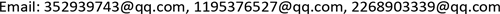MATLAB仿真，太阳影子定位，分层搜索，单目标优化, MATLAB Simulation Sun Shadow Localization Hierarchical Search Single Target Optimization1. 引言

2. 太阳影长变化模型2.1. 影长变化模型

α = arcsin ( sin φ sin D + cos φ cos D cosh ) (1)

sin D = sin 23 ∘ 2 6 ′ 37. 8 ″ sin σ (2)

δ = 279.9348 + d + 1.914827 sin d − 0.079525 cos d                                   + 0.019938 sin 2 d − 0.001620 cos 2 d (3)

d为一年中特定日期表示的角分数，有：

d = ( n − 1 ) × 360 365.242 (4)

h = 15 ( T − M ) − l o n g i t u d e (5)

T = t B − l o n g i t u d e + 120 15 − 8 (6)

M = 12 + 0.123570 sin d − 0.004289 cos d + 0.153809 sin 2 d + 0.060783 cos 2 d (7)

d的定义见式(4)。

2.2. 各因素对影长的影响

(I) 积日、直杆所在点经纬度和测量时间一定，直杆长度变化

t B = 12 , φ = 39.91 ∘ , l o n g i t u d e = − 116.39 ∘ , n = 295 。

(II) 积日、直杆所在点经度值和测量时间一定，直杆所在点纬度值变化

t B = 12 ; φ = 0 ∘ , 15 ∘ , 30 ∘ , 45 ∘ , 60 ∘ ; l o n g i t u d e = − 116.39 ∘ ; n = 295

(III) 积日、直杆所在点纬度值和测量时间一定，直杆所在点经度值变化

t B = 12 ; φ = 39.91 ∘ ; l o n g i t u d e = − 20 ∘ , − 30 ∘ , − 60 ∘ , − 80 ∘ , − 100 ∘ ; n = 295

(IV) 直杆所在点经纬度值和测量时间一定，积日变化

t B = 12 ; φ = 39.91 ∘ ; l o n g i t u d e = − 116.39 ∘ ; n = 40 , 100 , 170 , 230 , 290

η = n x i a − n min n x i a = 173 − 172 173 ≈ 0.578 % (8)

(V) 直杆所在点经纬度值和积日一定，测量时间变化

3. 太阳影子定位模型3.1. 定位模型的建立

l i = x i 2 + y i 2 (9)

| H max − H min | < 0.0005 (10)

3.2. 定位模型的求解

{ n = 108 t B i = 14.7 : 0.05 : 15.7 (11)

Latitude and longitude values that meet the condition

1−4−101
2−3−104
3−2−106
417−111
518−110
619−109
721−106

0.1474%~2.3646%，说明所求地点的经度值与海南经度范围的误差值很小，并且问题二题干中说明此直杆固定在水平地面上，则在误差允许范围内，本文将潜在地点二定位于中国海南省，并且综合两地多组杆长的数据可以得出问题二中的直杆长约为2.1 m。

4. 模型检验

Comparison of measured data with model result

9:301.88211.85261.57%
10:471.28691.26052.37%
12:101.11271.09431.65%
14:301.91481.93090.84%
16:003.56873.64832.23%

5. 稳定性分析

Stability analysis of latitud

10:004.46484.64214.29753.85%
12:003.67323.83343.52104.25%
14:005.11455.30954.93113.69%

6. 总结